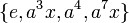# Subgroup structure of semidihedral group:SD16

Jump to: navigation, search

## Contents

This article gives specific information, namely, subgroup structure, about a particular group, namely: semidihedral group:SD16.
View subgroup structure of particular groups | View other specific information about semidihedral group:SD16

We are interested in the group$G = SD_{16}$, the semidihedral group:SD16, given by the presentation:$\langle a,x \mid a^8 = x^2 = e, xax^{-1} = a^3 \rangle$

where$e$ denotes the identity element.

The group has 16 elements:$\{ e, a, a^2, a^3, a^4, a^5, a^6, a^7, x, ax, a^2x, a^3x, a^4x, a^5x, a^6x, a^7x \}$

## Tables for quick information

### Table classifying subgroups up to automorphisms

FACTS TO CHECK AGAINST FOR SUBGROUP STRUCTURE: (group of prime power order)
Lagrange's theorem (order of subgroup times index of subgroup equals order of whole group, so all subgroups have prime power orders)|order of quotient group divides order of group (and equals index of corresponding normal subgroup, so all quotients have prime power orders)
prime power order implies not centerless | prime power order implies nilpotent | prime power order implies center is normality-large
size of conjugacy class of subgroups divides index of center
congruence condition on number of subgroups of given prime power order: The total number of subgroups of any fixed prime power order is congruent to 1 mod the prime.

Automorphism class of subgroups List of subgroups Isomorphism class Order of subgroups Index of subgroups Number of conjugacy classes (=1 iff automorph-conjugate subgroup) Size of each conjugacy class (=1 iff normal subgroup) Total number of subgroups (=1 iff characteristic subgroup) Isomorphism class of quotient (if subgroup is normal) Subnormal depth (if proper and normal, this equals 1) Nilpotency class
trivial subgroup$\{ e \}$ trivial group 1 16 1 1 1 semidihedral group:SD16 1 0
center of semidihedral group:SD16$\{ e, a^4 \}$ cyclic group:Z2 2 8 1 1 1 dihedral group:D8 1 1
non-normal order two subgroups of semidihedral group:SD16$\{ e, x \}, \{ e, a^2x \}, \{ e, a^4x \}, \{ e, a^6x\}$ cyclic group:Z2 2 8 1 4 4 -- 3 1
derived subgroup of semidihedral group:SD16$\{ e, a^2, a^4, a^6 \}$ cyclic group:Z4 4 4 1 1 1 Klein four-group 1 1
non-normal order four cyclic subgroups of semidihedral group:SD16$\{ e, ax, a^4, a^5x \}$,$\{ e, a^3x, a^4, a^7x \}$ cyclic group:Z4 4 4 1 2 2 -- 2 1
Klein four-subgroups of semidihedral group:SD16$\{ e, x, a^4, a^4x \}$,$\{ e, a^2x, a^4, a^6x \}$ Klein four-group 4 4 1 2 2 -- 2 1
Z8 in SD16$\langle a \rangle$ cyclic group:Z8 8 2 1 1 1 cyclic group:Z2 1 1
D8 in SD16$\langle a^2, x \rangle$ dihedral group:D8 8 2 1 1 1 cyclic group:Z2 1 2
Q8 in SD16$\langle a^2, ax \rangle$ quaternion group 8 2 1 1 1 cyclic group:Z2 1 2
whole group all elements semidihedral group:SD16 16 1 1 1 1 trivial group 0 3
Total (10 rows) -- -- -- -- 10 -- 15 -- -- --